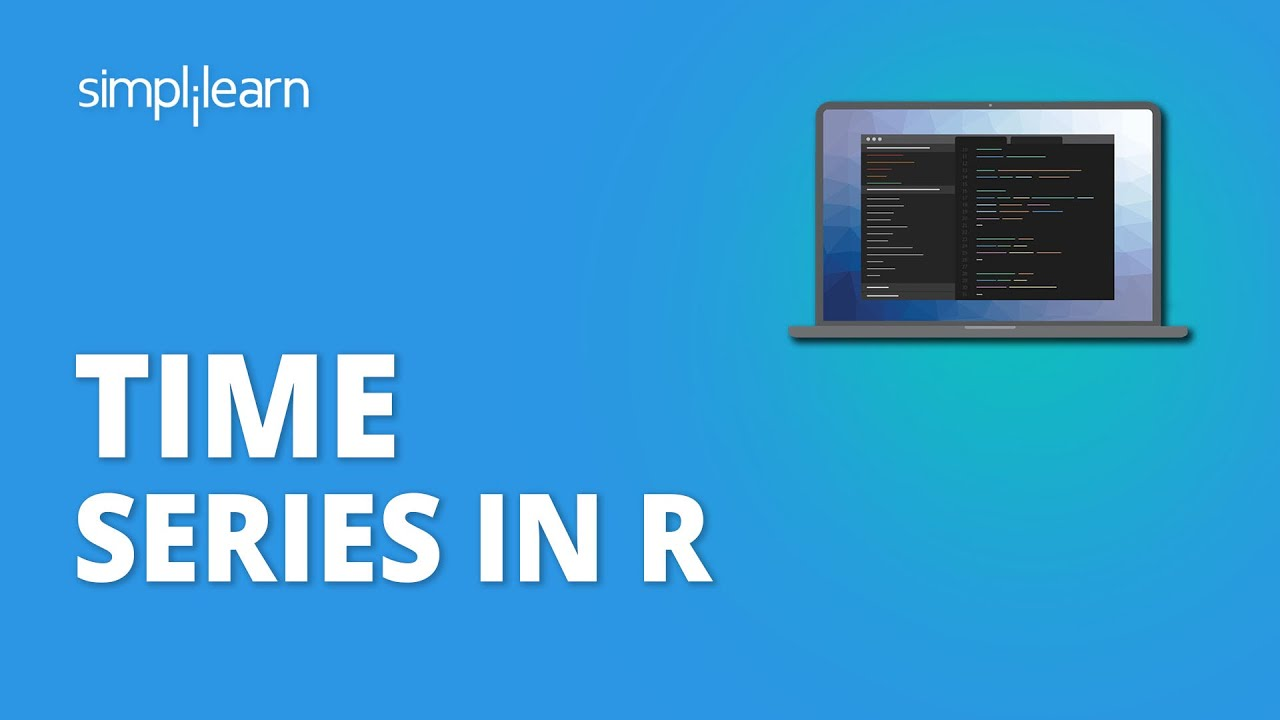# Time Series In R | Time Series Analysis In R Step By Step | R ProgrammingTime Series analysis is a standard machine learning problem. We'll perform Time Series Analysis in R. It is a hands-on project where we will use time-series energy data. We will understand how techniques such as time-based indexing, resampling, and rolling window can help us explore electricity demand variations and renewable energy supply over time.

Time Series analysis is a standard machine learning problem. In this video, we'll perform Time Series Analysis in R. It is a hands-on project where we will use time-series energy data. We will understand how techniques such as time-based indexing, resampling, and rolling window can help us explore electricity demand variations and renewable energy supply over time. Let's get started.

## Data Cleaning in R for Data Science

A data scientist/analyst in the making needs to format and clean data before being able to perform any kind of exploratory data analysis.

## R For Data Science Full Course | Data Science With R Full Course |Data Science Tutorial

Learn the essential concepts in data science and understand the important packages in R for data science. You will look at some of the widely used data science algorithms such as Linear regression, logistic regression, decision trees, random forest, including time-series analysis. Finally, you will get an idea about the Salary structure, Skills, Jobs, and resume of a data scientist.

## Data Science With R Training in Hyderabad | Data Science Courses in Hyderabad

Best Data Science With R Training in Hyderabad - We Provides Best Data Science Certification Courses in Hyderabad offering extensive Data Science With R Training by Data scientists. Enrol Today!